## FORM 3 PROBABILITY – MATHEMATICS TESTS

FORM 3 PROBABILITY – MATHEMATICS TESTS

1. .A bag contains 7 RED sweets, 5 GREEN sweets and 8 BLUE sweets. One sweet is taken out. What is the probability that the sweet is red or green?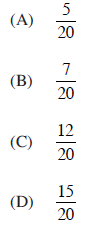2. These coins are in a box. One coin is taken out at random.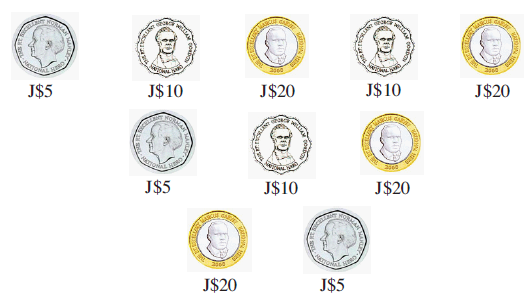What is the probability that it is a J\$20 coin?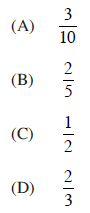3. The table shows the distribution of the ages of 25 students.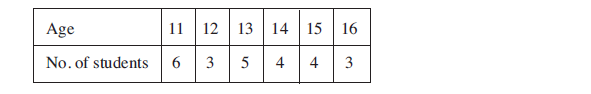What is the probability that a student chosen at random is AT LEAST 13 years old?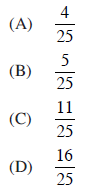4. In a box, there are 3 white, 4 red and 2 blue marbles. What is the probability that a marble taken at random is NOT blue?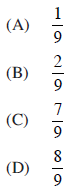5. A fair die is rolled twice. What is the probability of obtaining two sixes?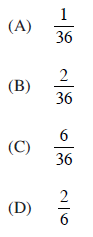Question 1 of 5